• 2021-06-17 11:31:58

Access如何制作复杂报表

何制作复杂报表——利用excel输出复杂报表

在读这篇文章以前首先要提醒大家，Access 本身的报表也具有很强的实用性和强大的功能，只有当你发掘了其本身全部的功能却仍不能满足你对报表的特殊要求时才请使用 Excel 输出报表。很明显，使用 Excel 输出报表第一个弱点就是必须同时打开 Access 和 Excel (当然，你得在一台机器上同时安装 Access 和 Excel)，当然如果你和我一样拥有工作在 966MHz 上的 CPU 和 256M 内存以及大容量硬盘时，那就不必考虑了，继续吧。

首先找到 VBA 编程界面并找到"工具"菜单并单击"引用..."菜单项，在弹出的"引用"窗体中找到"现有引用"列表，在名为"Microsoft Excel x.0 Object Library"的项目前的方框内打钩。

其次，你要做好一张空白的 Excel 电子表格，设定好显示字体、边框、打印纸张等等并将其存盘。(以下示例中该表格名为 "temp.xls" )当然啦，直接使用空白的 Excel 文件也是可以的，不过你要编程自动生成表格边框、背景颜色、单元格格式等一些内容，这些涉及到 Excel 编程，我以后会在 Excel 专栏里详细叙述，这里就不多说了。

然后你就可以在 Access 里面制作你自己的打印预览窗体了，在窗体中安排 2 个命令按钮，在名为预览的按钮的 click 事件里输入以下代码：

Private Sub ExcelPreview_Click()

Dim xlApp As Excel.Application

Dim xlBook As Workbook, xlSheet As Worksheet

Set xlApp = CreateObject("Excel.Application")

Set xlApp = New Excel.Application

xlApp.Visible = True

Set xlBook = xlApp.Workbooks.Open("c:\reprot\temp.xls")

Set xlSheet = xlBook.Worksheets(1)

xlSheet.Cells(3, 1) = "制表日期：" + "12" + " 月"

'以上只更改了一个单元格内的数据，可根据需要无限次加入

xlBook.Save

xlSheet.PrintPreview    '如果是要打印，只要把 PrintPreview 改为 PrintOut

xlBook.close

xlApp.Quit

End Sub

OK，然后就可以单击该按钮看看生成的表格如何了。

本文作者:相关阅读:

asp中静态页面实现方法

编程创建 SQL Server 数据库

Vista 修改注册表全面禁用控制面板

JavaScript 代码压缩工具小结

wordpress之js库集合研究介绍

Vfloppy通过CD光盘安装Linux到硬盘运行

TD的noWrap属性注意事项

JS 参数传递的实际应用代码分析

Red Hat Enterprise Linux 4+Nginx 0.7.47+PHP5.2+MYSQL5.0+Memcache+eAccelerator收

JScript脚本技术基础

javascript轻松控制表格列样式的脚本代码

PHP 5.0 Pear安装方法

Windows7操作系统的电源设置功能

更多相关内容
• 多功能报表 一、报表概述 此报表可配合趋势功能实现历史数据的追溯，查询所有数据整个生命周期的数据变化情况，以数据报表的形式显示出来。 二、报表功能介绍 此报表可根据趋势的时间范围来进行数据显示，...

# 多功能报表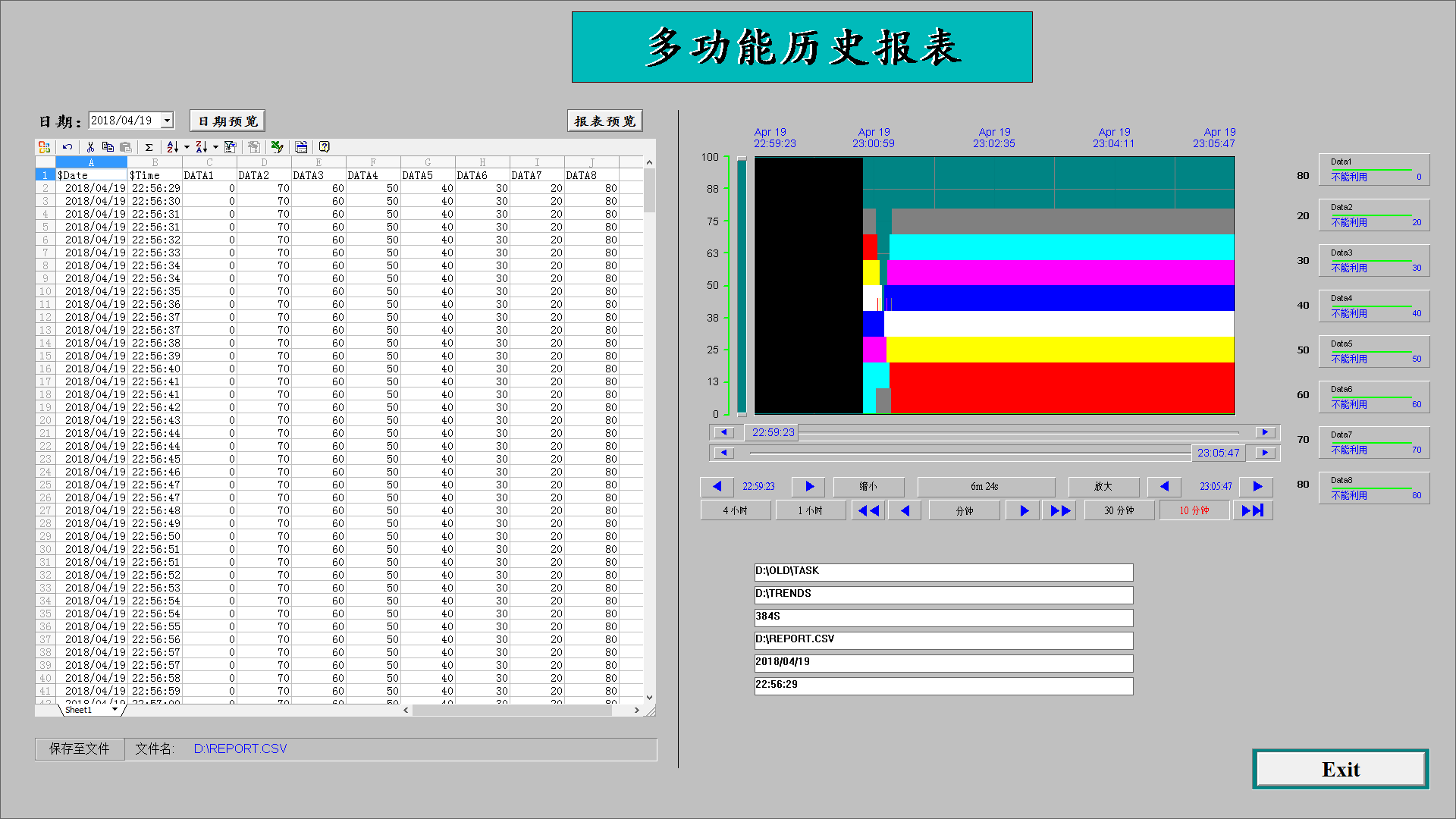一、      报表概述

此报表可配合趋势功能实现历史数据的追溯，查询所有数据整个生命周期的数据变化情况，以数据报表的形式显示出来。

二、      报表功能介绍

此报表可根据趋势的时间范围来进行数据显示，显示时间范围内每秒的数据大小。可对表格里面的数据作相应的动作，如：“撤销”、“剪切”、“复制”、“粘贴”、“求和运算”、“排序”、“自动筛选”、“刷新表格”、“导出为Excel文档”、“格式编辑”等。

三、      报表延伸

此报表的导出功能可根据一定的排版格式和的背景颜色来更加专业的化定制业主需求。可单独作为各种生产数据存储和查询的工具，配合SQL Server等数据库对数据进行搜索。

相关的视频学习教程可进入下面链接自主学习：

Wonderware- Intouch 利用 Excel 控件制作报表，功能强大

展开全文控件
• 手把手教你利用VBA+excel制作 IFIX 报表 可完美移植到VB6.0。 正在的手把手，一步一图，代码详解！ 其中主要讲述如何判断文件、数据文件的存在、建立、修改数据、读入、写入、删除。并如何利用EXCEL模块建立报表，...
• 我自己写的关于如何利用C#和Excel制作高级报表的例子
• 利用VB操作EXCEL来生成复杂的报表 vb操作EXCEL文件_编程技术收藏
• Excel动态数据报表制作

# 一、基本概念

1.表格组成：工作簿-工作表-行/列-单元格
2.功能区
（空闲时可逐一进行了解）鼠标悬停在功能区某一按钮上即会出现该功能注释# 二、基础操作

• 源数据备份
拿到一份数据之后，第一步操作进行备份，以免对数据造成不可还原的修改。
操作：选中源数据工作表-【右键】选择【移动或复制工作表】-选中【移至最后】并勾选【创建副本】-选中副本-【右键】【重命名】为“源数据备份”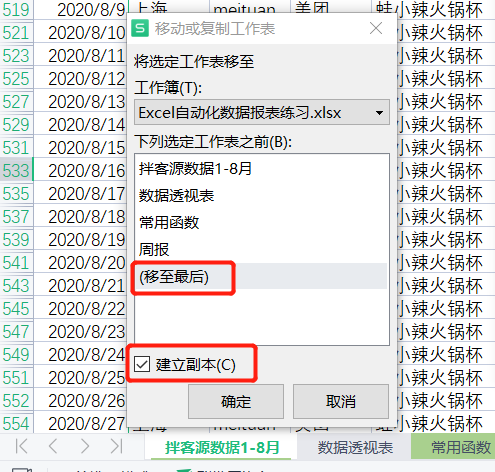# 三、理解数据

## 1.数据量级

• 选中整行：整个数据一共24列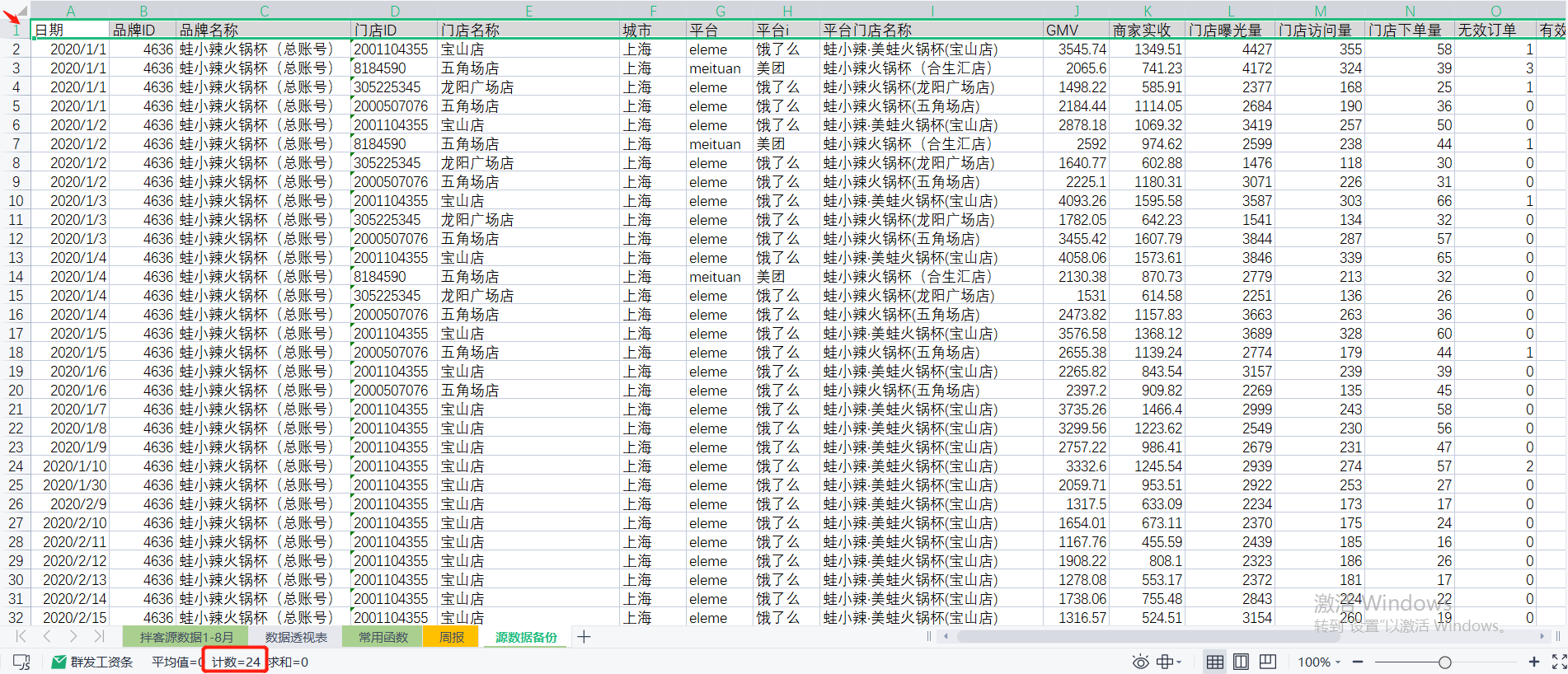• 选中整列：整个数据一共562行，除去表头数据实际一共521行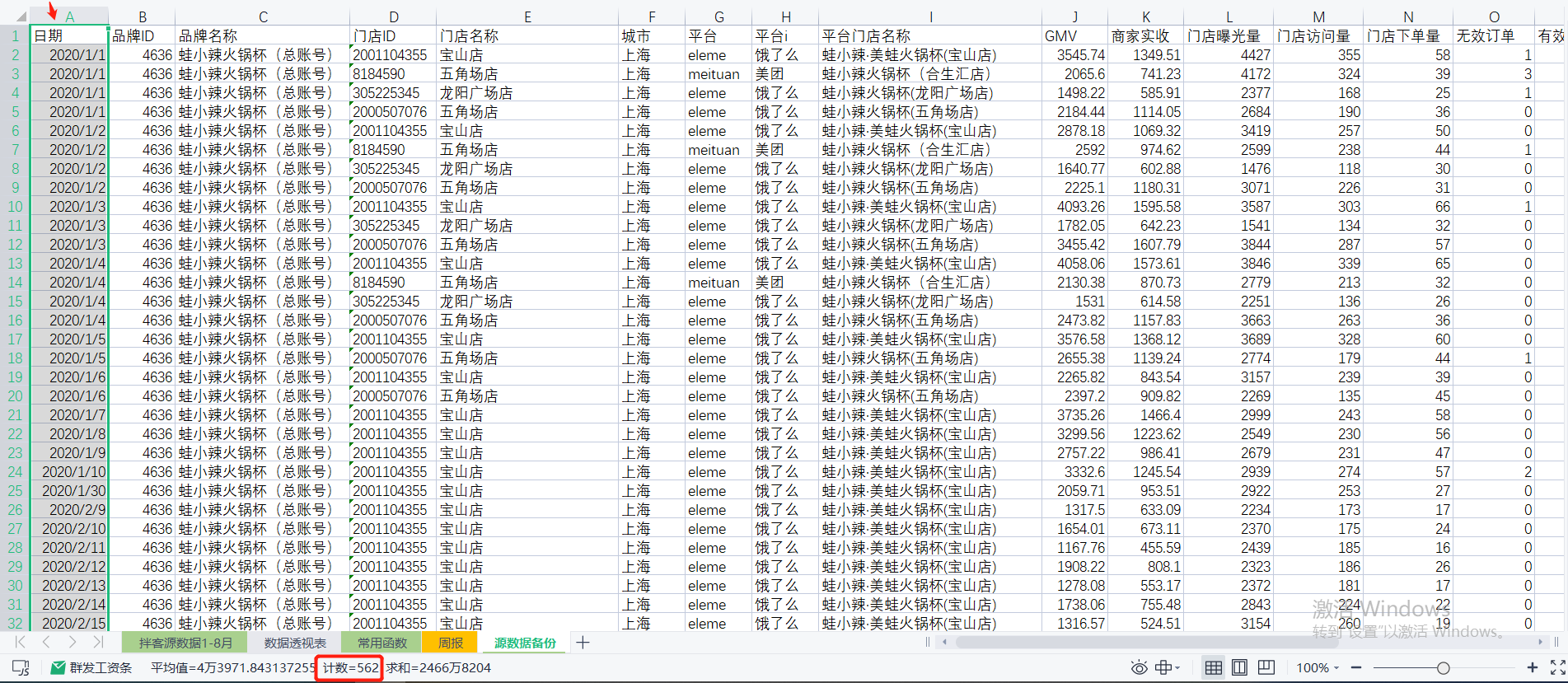## 2.数据类型

注:该报表使用的数据为线上外卖门店的数据

• 日期:指的是一家外卖门店当天所有营业额所归属的日期；
• 品牌ID:相当于品牌身份证号
• 品牌名称:门店所在品牌名称
Ctrl+Shift+L快速筛选，可以快速了解门店品牌数、排序、筛选等；
• 门店ID:相当于门店的身份证号
• 门店名称
• 城市
• 平台:平台的拼音格式
• 平台i:平台的中文格式
• 平台门店名称
注：同样一家店，存在不一样的格式（如:拌客干拌麻辣烫(武宁路店)→拌客干拌麻辣烫（武宁路店）→拌客·干拌麻辣烫(武宁路店)，以增加空格、点的形式区分店名），原因是该门店存在关店重开的情况，每次关店重开后会更换平台门店名称以此与之前的门店做区分，更方便的收集数据。
• GMV:全称为GrossMerchandiseVolume即商品交易总额，是一段时间内的成交总额的意思，多用于电商行业，一般包含拍下未支付订单金额（未刨除各项补贴的表面金额）。
• 商家实收:去除平台补贴、商家补贴、红包、满减、配送费、平台抽成等等后，当天实际可以进入银行卡/平台账户收入里的金额
• 门店曝光量:指的是该门店在外卖平台被用户看到的次数
• 门店访问量:用户进入该门店的次数
• 门店下单量
• 无效当订单
• 有效订单
• 曝光人数：对应去重的曝光量
• 进店人数：对应去重的访问量
• 下单人数：对应去重的下单量
• cpc总费用:CPC(Cost Per Click)每产生一次点击所花费的成本。在这个报表中cpc总费用指的是当天广告投放的总花费
• cpc曝光量:广告投放为门店带来的曝光量
• cpc访问量:广告投放为门店带来的访问量
• 商户补贴:商家补贴金额
• 平台补贴：平台补贴金额

## 3.数据含义

### 环比

环比=(本期数/上期数)/上期数=本期数/上期数-1


1)年环比

2020年环比=(2020年数据-2019年数据)/2020年数据=2020年数据/2019年数据-1


2)月环比

2020年7月环比=2020年7月数据/2020年6月数据-1


3)日环比

2020年7月1日环比=2020年7月1日数据/2020年6月30日数据-1


### 同比

同比=(本期数-同期数)/本期数=本期数/同期数-1


1)月同比

2020年7月同比=2020年7月数据/2019年7月数据-1


2)日同比

2020年7月1日的月同比=2020年7月1日数据/2020年6月1日数据-1
2020年7月1日的周同比=2020年7月1日数据/2020年6月24日数据-1


# 四、常用函数

1.sum：可以对整行、整列、一个区域进行求和

2.sumif：单条件求和

sumif(range,criteria,[sum_range])
sumif(条件判断所在的区域,条件,[用来求和的数值区域])


3.sumifs：多条件求和

sumifs(sum_range,[criteria_range1],[criteria1],[criteria_range2],[criteria2],..)
sumifs(用来求和的数值区域,条件1判断所在的区域1,条件1,条件2判断所在的区域1,条件2,...)


例：求整月美团平台的GMV

=sumifs(GMV列,平台列,"美团",日期列,">="&每月第一天,日期列,"<="&每月最后一天)


注：条件参数直接引用单元格或者使用函数不需要英文双引号，若使用如"美团"这样的字符串做条件参数，需要加英文双引号；以及大于等于号也需要添加英文双引号，并且使用&才能与后面的条件值相连。

4.subtotal：根据筛选求和、平均等等（11个函数,以数字区分，9为sum函数）

subtotal(function_num,ref1,[ref2],...)
subtotal(指定函数，选择区域1，[选择区域2],...)


例如：

subtotal(9,GMV列)=sum(GMV列) 仅当全选时该等式成立。


5.Year:提取日期的年，以数字格式显示

YEAR(serial_number) YEAR(日期)


6.Month:提取日期的月

MONTH(serial_number) MONTH(日期)


7.DAY:提取日期的天

DAY(serial_number) DAY(日期)


8.DATE:组合年月日

DATE(year,month,day) DATE(代表年份的数值，代表月份的数值，代表日份的数值)


与YEAR()、MONTH()、DAY()组合，可用来求得上一年、上月、上周、昨天、下一年、下个月、下周、明天等对应日期。例如：

每个月的第一天=DATE(year(日期),month(日期),1)；
每个月的最后一天=下个月的第一天-1=DATE(year(日期),month(日期)+1,1) -1


注：不要使用excel里的日期格式存储日期，建议使用字符串形式存储日期，否则将表格导入数据库会出现其他问题

8.if：逻辑判断

if(logical_test,value_if_true,[value_if_false])
if(逻辑比较条件,结果成立时返回的值,[结果不成立时返回的值])
[value_if_false]:该参数选填，没有该参数时，返回False


例：利用if函数嵌套，判断A、B是否为0

if(A=0,if(B=0,"AB都为0","A等于0，B不等于0"),if(B=0,"A不等于0，B等于0","AB都不等于0"))


9.vlookup：连接匹配数据

VLOOKUP(lookup_value,table_array,col_index,[range_lookup])
VLOOKUP(要查找的数据,要查找的位置和要返回的数据的区域,要返回的数据在区域中的列号,返回近似匹配或精确匹配-1/true或0/false)

• 要查找的位置必须在区域的第一列，否则会报错
• VLOOKUP只会返回它查找到的第一个值
• VLOOKUP的模糊匹配非通常的模糊匹配，使用VLOOPUP模糊匹配文本类型数据时可以配合通配符使用。
通配符：
*:代替不定数量的字符
？:(英文输入状态下)代替一个字符


配合通配符进行模糊匹配（第三个参数为0）：

返回以A开头的数据对应的第二列的值=VLOOKUP("A*",区域,2,0)
返回以A开头的三个字符对应的第二列的值=VLOOKUP("A??",区域,2,0)


10.match：查找数值在区域中的位置

MATCH(lookup_value,lookup_array,[match_type])
MATCH(查找项,查找区域,0)


例：返回A在第几行

=MATCH("A",A所在列,0)


返回A在第几列

=MATCH("A",A所在行,0)


11.index：根据区域的位置返回数值

INDEX(array,row_num,column_num)
INDEX(区域，行号，列号)


12.match、index一起使用：自动根据列名查找数据
制作报表时，可以利用match函数在源数据中找到报表表头对应的数据列号或行号，结合Index函数找到对应的列/行进行后续计算。
例：图一为源数据表，图二为数据报表，根据表头信息在源数据中找到对应数据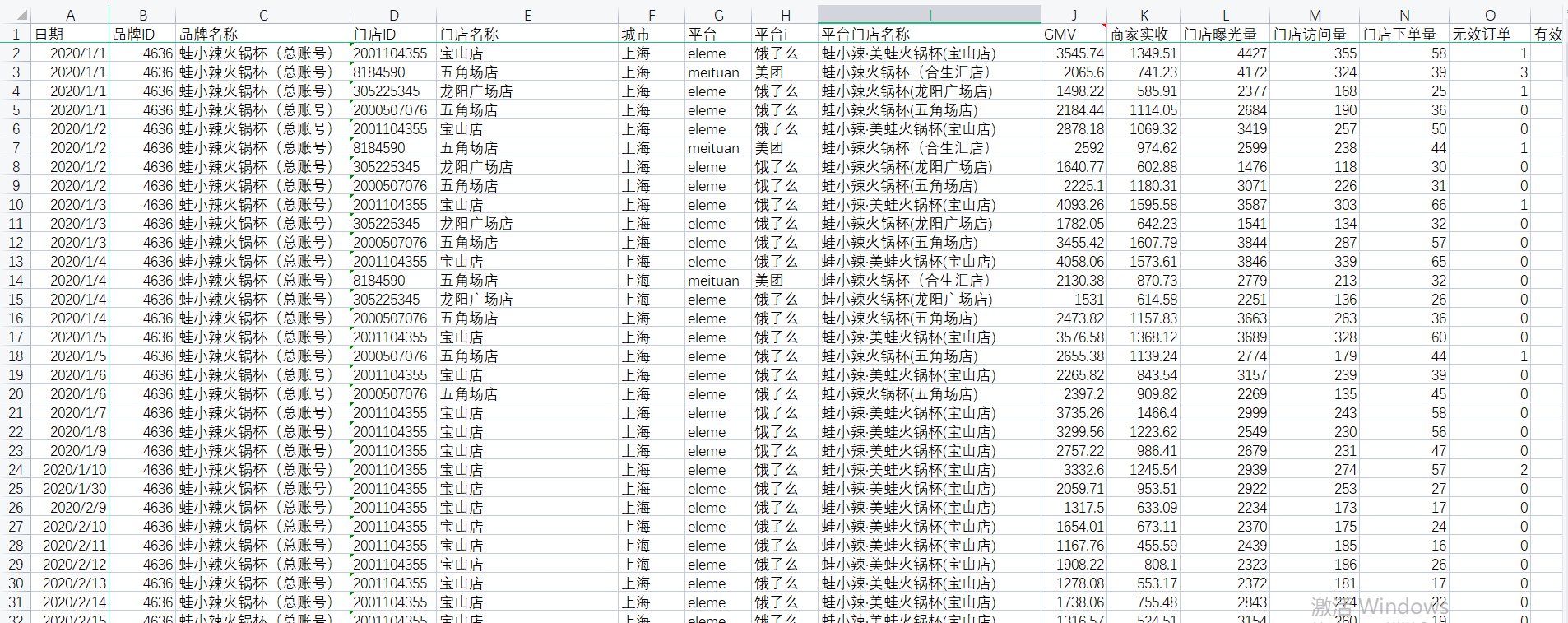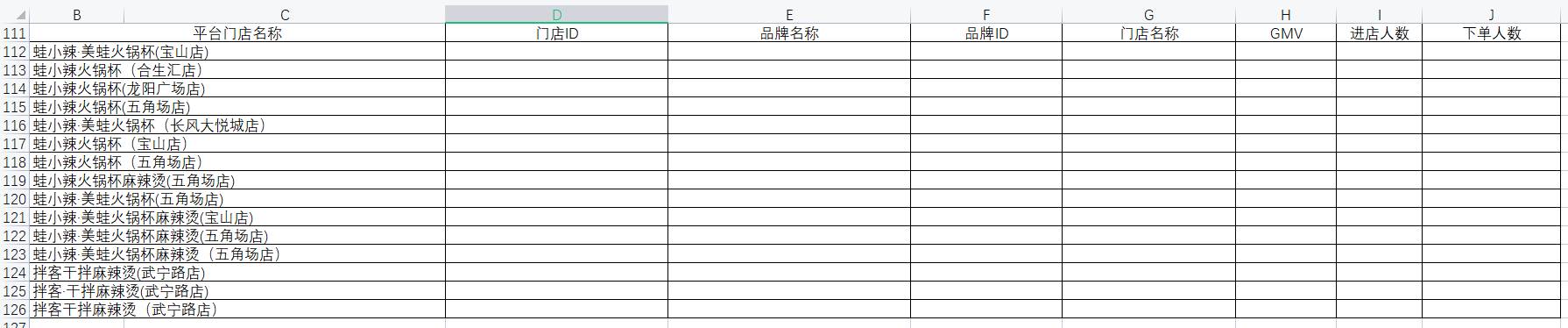过表头查找【蛙小辣·美蛙火锅杯(宝山店)】即【B112】单元格对应的门店ID：

• 因为要查找的数据在源数据表中，所以INDEX函数第一个区域参数为源数据所有列，即
'源数据表'!A:X    （!表示非本工作表）

• 【蛙小辣·美蛙火锅杯(宝山店)】的门店ID与该门店的平台店名称在同一行，所以查找门店ID的行号等同于查找【蛙小辣·美蛙火锅杯(宝山店)】在源数据表的行号。【蛙小辣·美蛙火锅杯(宝山店)】在【平台店名称】列，即I列，使用MATCH定位行号，即
MATCH(B112,'源数据表'!I:I,0)

• 【蛙小辣·美蛙火锅杯(宝山店)】门店ID的列，即查找【门店ID】即【D111】单元格在源数据的列号，【门店ID】在第一行，使用MATCH定位列号，即
MATCH(D111,'源数据表'!1:1,0)

• 完整函数格式为：
=INDEX('源数据表'!A:X,MATCH(B112,'源数据表'!I:I,0),MATCH(D111,'源数据表'!1:1,0))


编写好单个函数后，希望通过下拉、右拉可以自动查找对应的其他值。但是，下拉后会出现部分匹配不到数据的情况。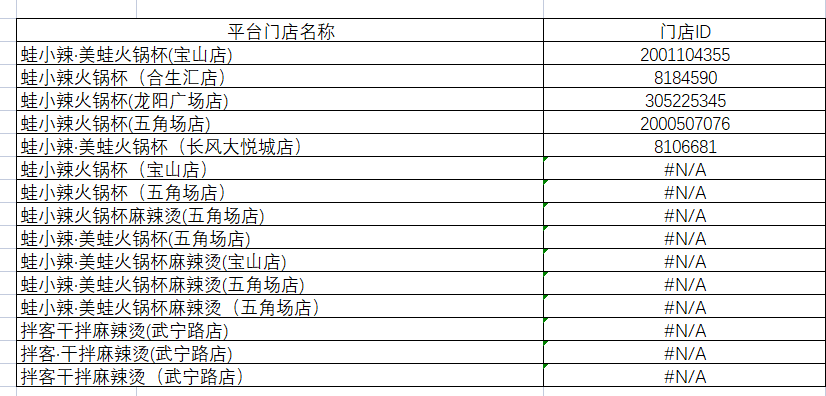因为下拉的同时，单元格的选中区域【蛙小辣·美蛙火锅杯(宝山店)】、【门店ID】等会依次向下移动，因此需要固定部分选中区域，让我们在下拉、右拉的同时保持不变。使用美元符号"$"进行锁定操作。 1）源数据表各区域固定，均需锁住； 2）[B112]需根据下拉向下拉匹配其他平台门店名称数据，同时右拉时固定该列，因此只需锁定B列,即$B112；
3）[D111]需根据右拉匹配其他表头数据，同时下拉时固定该行，即 D$111  • 完整函数格式为： =INDEX('源数据表'!$A:$X,MATCH($B112,'源数据表'!$I:$I,0),MATCH(D$111,'源数据表'!$1:$1,0))  13.sumifs、match、index顶级用法：自动根据列名的数据和条件进行求和 INDEX函数中，当行号为0时，返回一整列，当列号为0时返回一整行，因此通过MATCH返回查找值在源数据表中时第几行/列，再通过INDEX定位整列区域，结合sumifs函数进行条件筛选求和计算。 GMV、进店人数、下单人数均用sumifs、match、index结合的方式计算。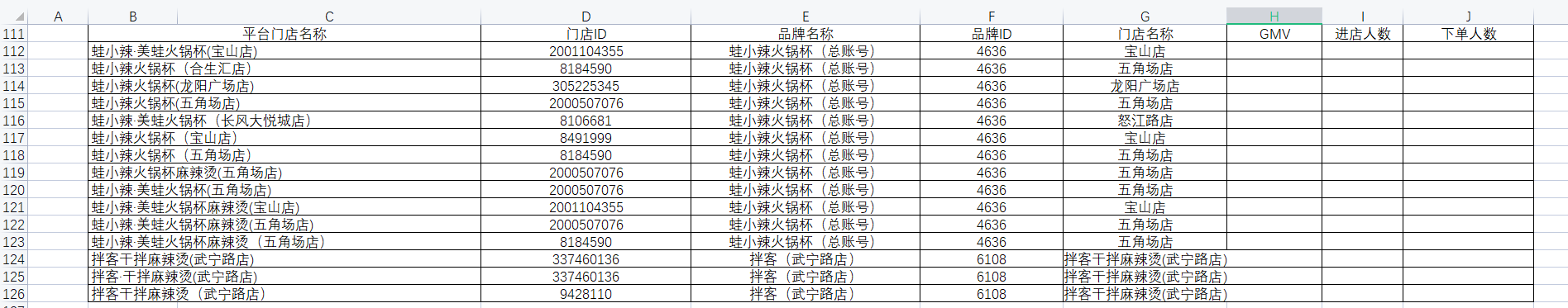求GMV • 利用INDEX、MATCH函数取得求和区域GMV列、取得条件判断区域平台店名称列： GMV列=INDEX('源数据表'!$A:$X,0,MATCH(H$111,'源数据表'!$1:$1,0))
平台店名称列=INDEX('源数据表'!$A:$X,0,MATCH($B$111,'源数据表'!$1:$1,0))

• 组合sumifs函数求出平台门店对应GMV总和
=SUMIFS(INDEX('源数据表'!$A:$X,0,MATCH(H$111,'源数据表'!$1:$1,0)),INDEX('源数据表'!$A:$X,0,MATCH($B$111,'源数据表'!$1:$1,0)),$B112)

• 通过向下拉动、向右拉动可自动得出门店下单人数总和、下单人数总和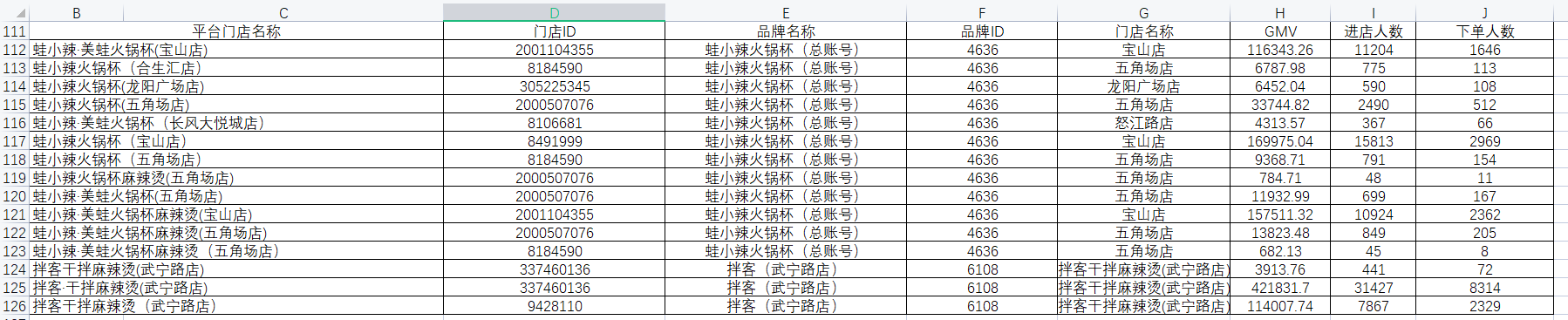# 五、自动化报表开发

## 1.报表框架

### 1)整体分布

一共分为四个部分：标题、小看板（含目标）、结果指标、过程指标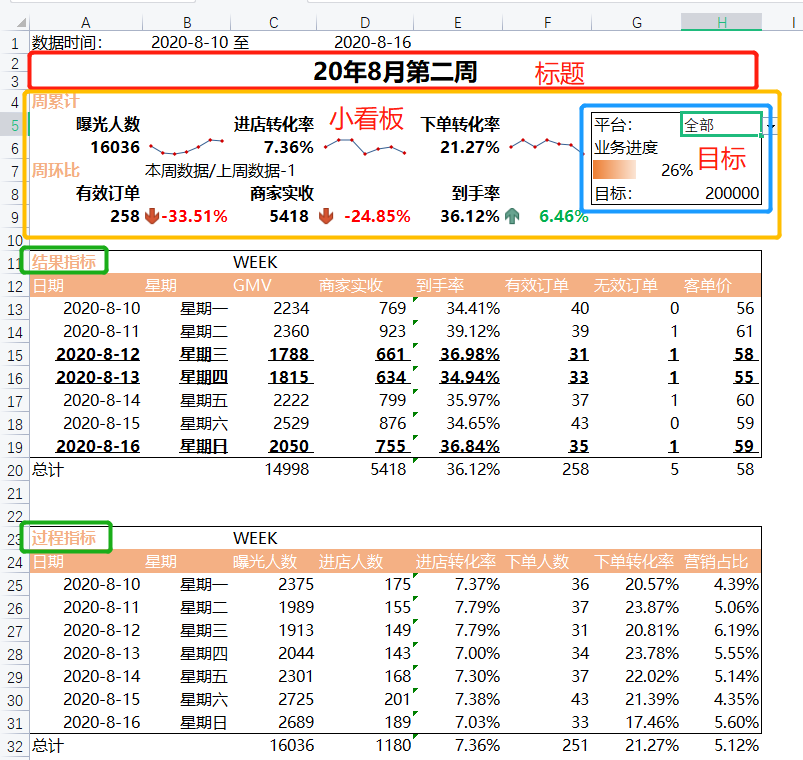### 2)时间信息

①日期
日期只需填写一个，其他日期均再次日期的基础上引用，可以极大提升效率，更改一个日期可以联动更新整个工作表的数据。
过程指标中的日期也是通过引用该日期实现。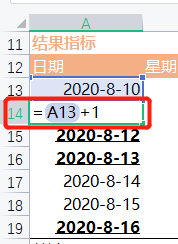②星期
同样引用上一个日期，更改格式即可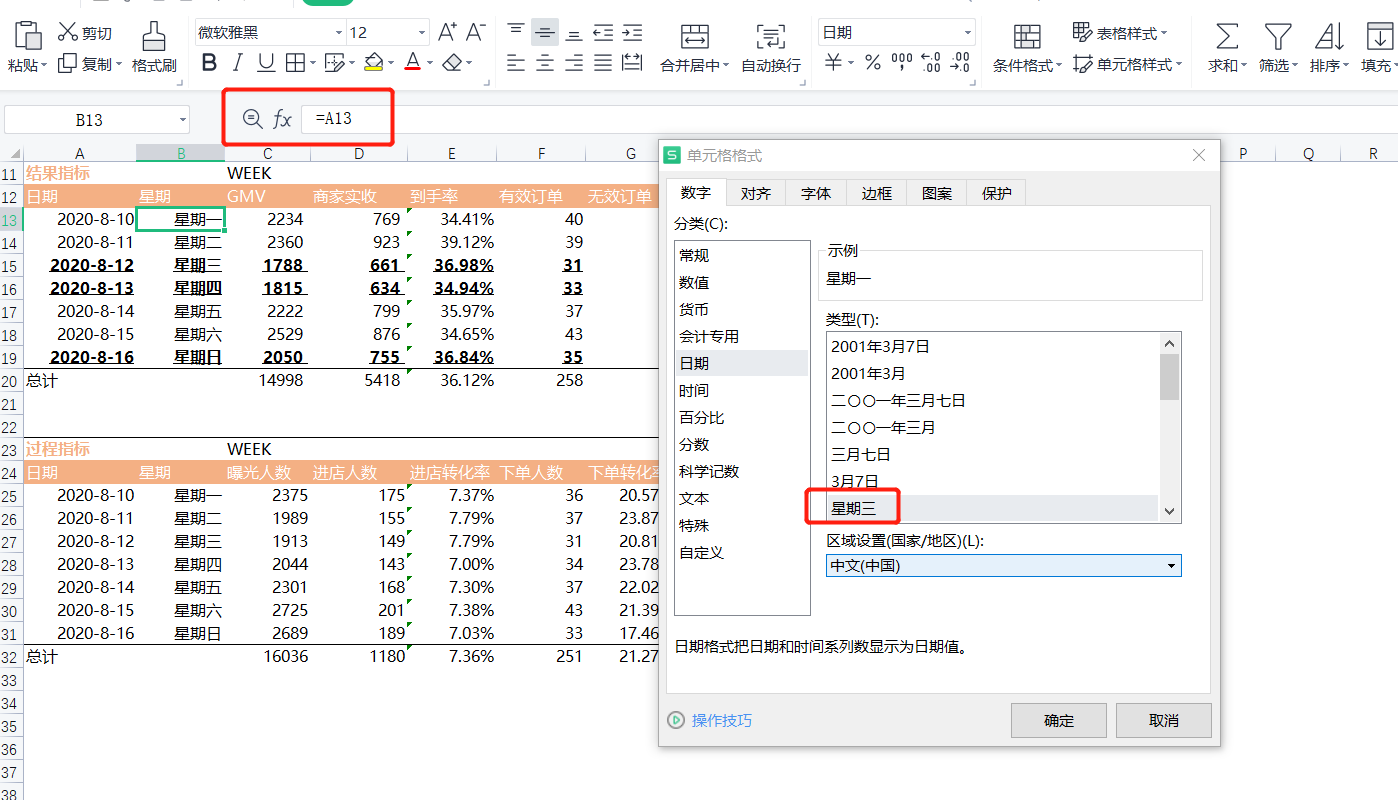③数据时间
同样进行日期的引用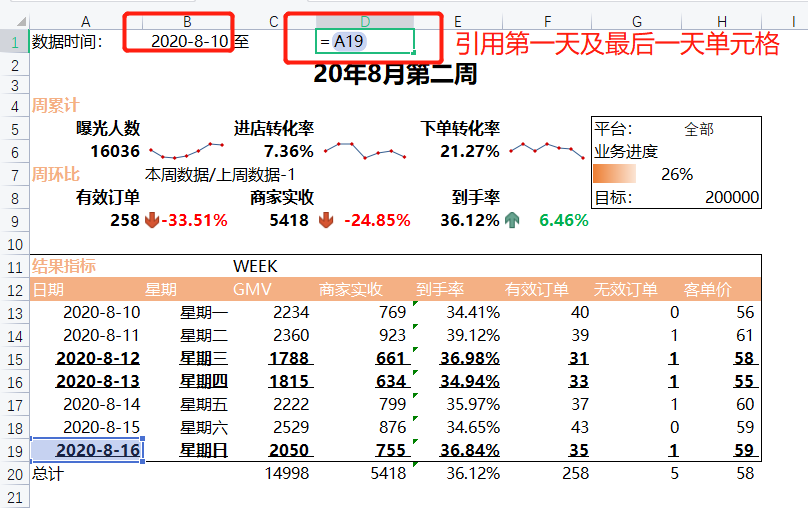## 2.指标梳理

### 1)结果指标

与商家收入的相关指数
GMV、商家实收、到手率(商家实收/GMV)、有效订单、无效订单、客单价(GMV/有效订单)

### 2)过程指标

商家经营情况的直观体现
曝光人数、进店人数、进店转化率(进店人数/曝光人数)、下单人数、下单转化率(下单人数/进店人数)、营销占比(cpc总费用/GMV)

## 3.联动筛选

1)下拉筛选器
在小看板中目标位置，设置了一个可供切换平台的筛选器，实际上它是一个条件验证，其他函数通过判断当前所选平台来计算对应数据。因此需要先设置该筛选器，后续判断一律引用该筛选器单元格，实现切换平台联动更新数据表。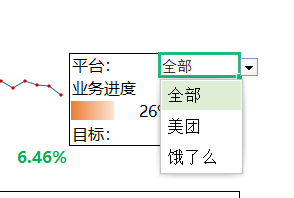对应操作：【数据】→【数据验证or数据有效性】→选择【序列】→输入【全部,美团,饿了么】注意使用英文逗号→【确定】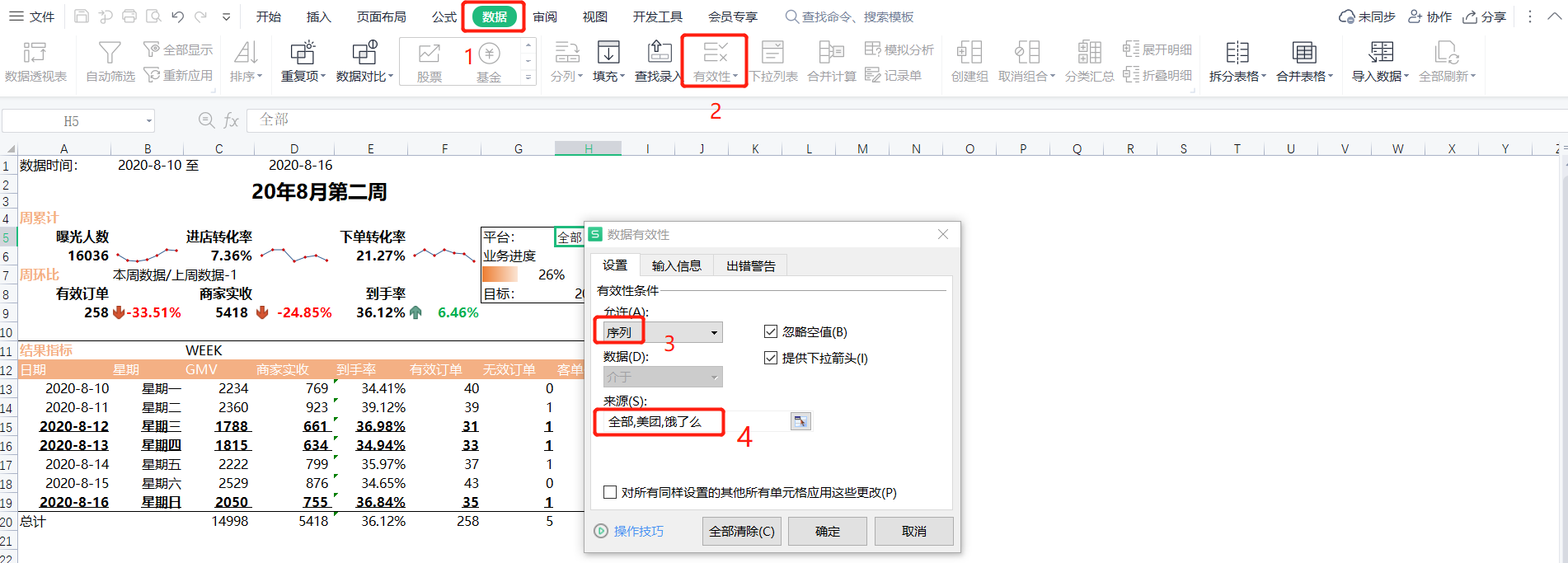## 4.条件求和

### 1)if、sumif、sumifs函数嵌套逻辑

求和GMV指标，需判断当前平台以及日期筛选。
if函数判断当前平台是全部、美团还是饿了么，当平台为全部时，无需进行平台筛选，使用sumif函数按日期筛选GMV求和即可；当平台为美团或饿了么时，需使用sumifs函数同时进行平台筛选及日期筛选GMV求和。

### 2)条件求和函数实现

if(平台=全部,sumif(日期列,日期,GMV列),sumifs(GMV列,日期列,日期,平台列,平台))


为了实现最大的灵活程度，使用index根据表头查找对应源数据列，只编写一此，通过向下向右拖拽即可自动填充其他求和项。

• 日期列
INDEX('源数据'!$A:$X,0,MATCH($A$12,'源数据表'!$1:$1,0))

• GMV列（求和列）
INDEX('源数据表'!$A:$X,0,MATCH(C$12,'源数据表'!$1:$1,0))  • 平台列 INDEX('源数据表'!$A:$X,0,MATCH("平台i",'源数据表'!$1:$1,0))  • 完整函数实现 =IF($H$5="全部",SUMIF(INDEX('源数据'!$A:$X,0,MATCH($A$12,'源数据表'!$1:$1,0)),$A13,INDEX('源数据表'!$A:$X,0,MATCH(C$12,'源数据表'!$1:$1,0))),SUMIFS(INDEX('源数据表'!$A:$X,0,MATCH(C$12,'源数据表'!$1:$1,0)),INDEX('源数据表'!$A:$X,0,MATCH($A$12,'源数据表'!$1:$1,0)),$A13,INDEX('源数据表'!$A:$X,0,MATCH("平台i",'源数据表'!$1:$1,0)),$H$5))  将该函数应用到过程指标中的【曝光人数】，只需将求和列中的GMV单元格修改为曝光人数对应的单元格即可，其他不变。 INDEX('源数据表'!$A:$X,0,MATCH(C$24,'源数据表'!$1:$1,0))

• 【曝光人数】完整函数实现
=IF($H$5="全部",SUMIF(INDEX('源数据表'!$A:$X,0,MATCH($A$24,'源数据表'!$1:$1,0)),$A25,INDEX('源数据表'!$A:$X,0,MATCH(C$24,'源数据表'!$1:$1,0))),SUMIFS(INDEX('源数据表'!$A:$X,0,MATCH(C$24,'源数据表'!$1:$1,0)),INDEX('源数据表'!$A:$X,0,MATCH($A$24,'源数据表'!$1:$1,0)),$A25,INDEX('源数据表'!$A:$X,0,MATCH("平台i",'源数据表'!$1:$1,0)),$H$5))

• 商家实收、有效订单、无效订单均可通过GMV指标右拉实现数据自动填充；
• 到手率=商家实收/GMV客单价=GMV/有效订单，这两个指标进行简单除法运算即可；
• 进店人数、下单人数均可通过曝光人数指标右拉实现数据自动填充；
• 进店转化率=进店人数/曝光人数下单转化率=下单人数/进店人数，这两个指标同样进行简单除法运算即可；
• 营销占比=cpc总费用/GMV。但是当前数据报表中没有展示cpc总费用的数据，需要额外进行计算，计算方式同求GMV的函数实现完全一致，只需将GMV单元格C12修改为"cpc总费用"(注意中文字符串需加上英文格式双引号)即可，再引用结果指标中已经计算好的GMV数据即可。完整函数实现如下：
=IF($H$5="全部",SUMIF(INDEX('源数据表'!$A:$X,0,MATCH($A$12,'源数据表'!$1:$1,0)),$A13,INDEX('源数据表'!$A:$X,0,MATCH("cpc总费用",'源数据表'!$1:$1,0))),SUMIFS(INDEX('源数据表'!$A:$X,0,MATCH("cpc总费用",'源数据表'!$1:$1,0)),INDEX('源数据表'!$A:$X,0,MATCH($A$12,'源数据表'!$1:$1,0)),$A13,INDEX('源数据表'!$A:$X,0,MATCH("平台i",'源数据表'!$1:$1,0)),$H$5))/C31


### 3)总计计算

• GMV、商家实收、有效订单、无效订单指标的总计，使用sum函数计算即可；
• 总计的到手率、客单价依旧使用简单除法计算；
• 曝光人数、进店人数、下单人数指标的总计，同样使用sum函数计算即可；
• 总计的进店转化率、下单转化率依旧使用简单除法计算；
• 总计的营销占比=一周的cpc总费用总计/一周的GMV总计。GMV总计可以从结果指标中引用C20单元格，cpc总费用总计需额外进行计算。计算一周的cpc总费用，只需要再计算一天cpc总费用函数的基础上，将单个的日期筛选修改为两个日期的大小判断，大于等于当周的第一天，小于等于当周的最后一天，通过引用日期列的开始日期单元格A13、结束日期单元格A19即可。逻辑如下
if(平台=全部,sumifs(cpc总费用列,日期列,">="&开始日期,日期列,"<="&结束日期),sumifs(cpc总费用列,日期列,">="&开始日期,日期列,"<="&结束日期,平台列,平台))

• cpc总费用总计完整函数实现
IF($H$5="全部",SUMIFS(INDEX('源数据表'!$A:$X,0,MATCH("cpc总费用",'源数据表'!$1:$1,0)),INDEX('源数据表'!$A:$X,0,MATCH($A$12,'源数据表'!$1:$1,0)),">="&$A13,INDEX('源数据表'!$A:$X,0,MATCH($A$12,'源数据表'!$1:$1,0)),"<="&$A19),SUMIFS(INDEX('源数据表'!$A:$X,0,MATCH("cpc总费用",'源数据表'!$1:$1,0)),INDEX('源数据表'!$A:$X,0,MATCH($A$12,'源数据表'!$1:$1,0)),"<="&$A13,INDEX('源数据表'!$A:$X,0,MATCH($A$12,'源数据表'!$1:$1,0)),"<="&$A19,INDEX('源数据表'!$A:$X,0,MATCH("平台i",'源数据表'!$1:$1,0)),$H$5))

• 总计的营销占比完整函数实现
=IF($H$5="全部",SUMIFS(INDEX('源数据表'!$A:$X,0,MATCH("cpc总费用",'源数据表'!$1:$1,0)),INDEX('源数据表'!$A:$X,0,MATCH($A$12,'源数据表'!$1:$1,0)),">="&$A13,INDEX('源数据表'!$A:$X,0,MATCH($A$12,'源数据表'!$1:$1,0)),"<="&$A19),SUMIFS(INDEX('源数据表'!$A:$X,0,MATCH("cpc总费用",'源数据表'!$1:$1,0)),INDEX('源数据表'!$A:$X,0,MATCH($A$12,'源数据表'!$1:$1,0)),"<="&$A13,INDEX('源数据表'!$A:$X,0,MATCH($A$12,'源数据表'!$1:$1,0)),"<="&$A19,INDEX('源数据表'!$A:$X,0,MATCH("平台i",'源数据表'!$1:$1,0)),$H$5))/C20


## 5.迷你图

小看板中周累计的曝光人数、进店转化率、下单转化率直接引用过程指标数据即可。
插入迷你图操作
【选中整周数据】→菜单【插入】→功能区【折线】→选中迷你图放置区域→【确定】→勾选【标记】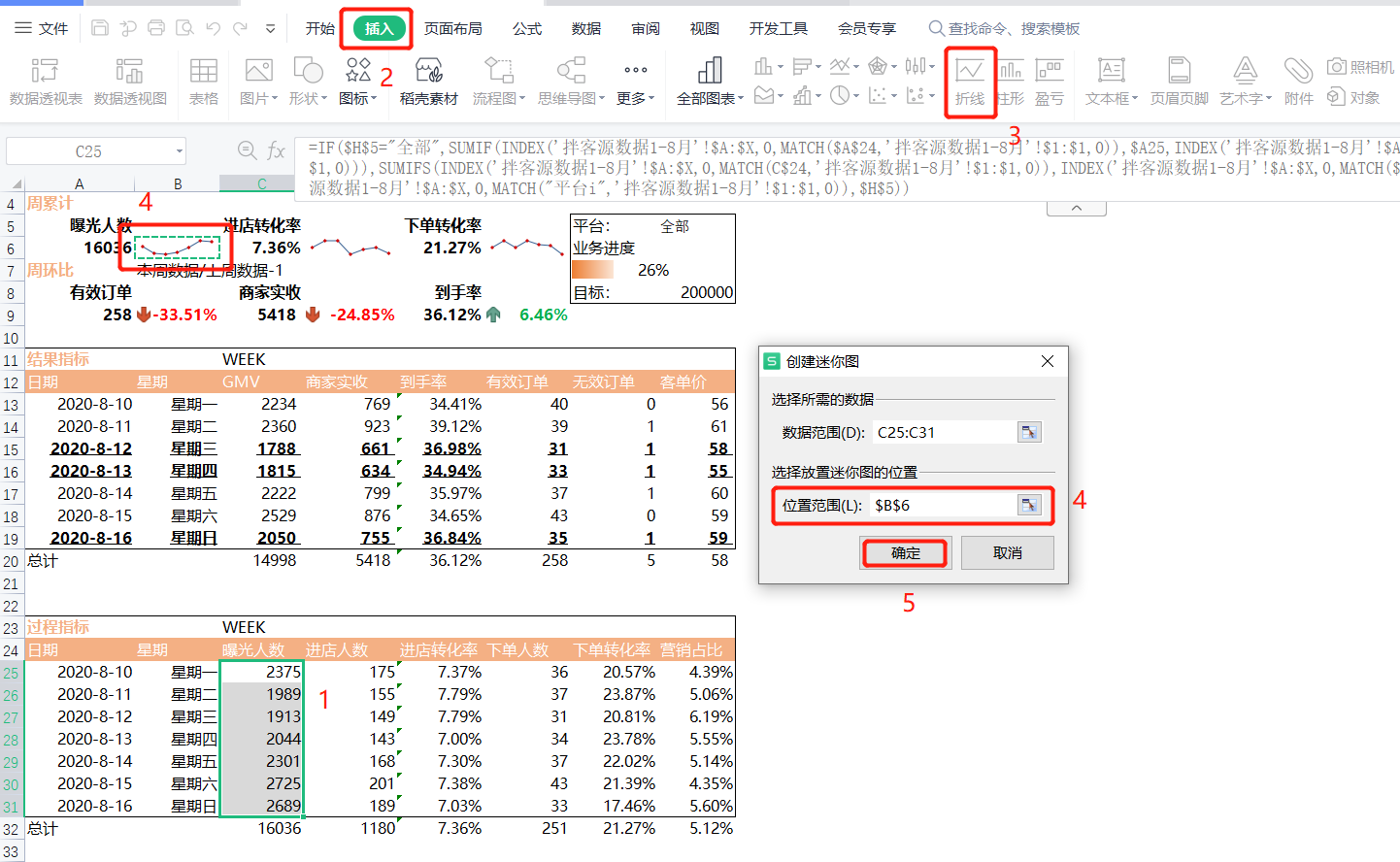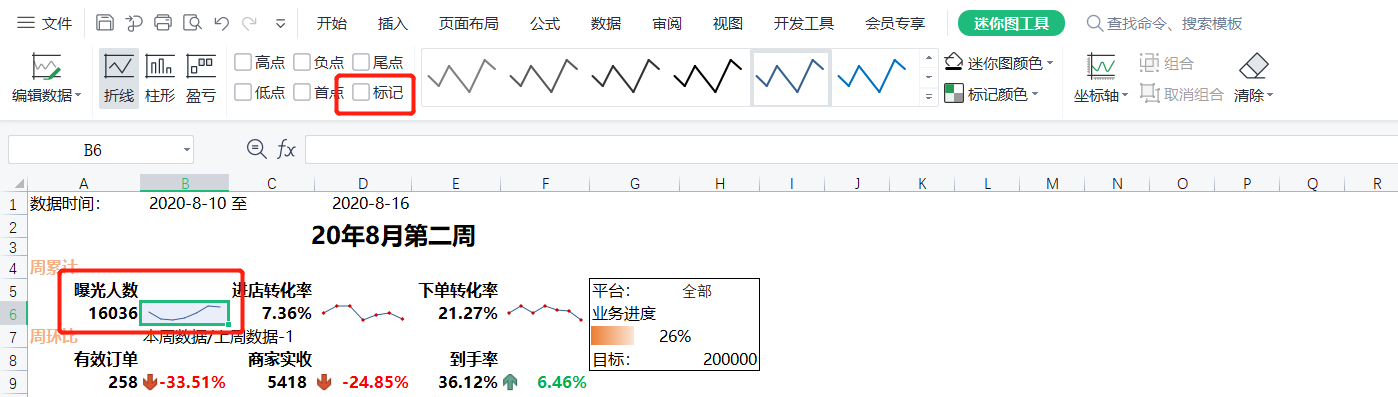## 6.同比/环比计算

周环比=本周数据/上周数据-1

小看板中周环比的本周有效订单、商家实收、到手率直接引用过程指标数据即可。
上周的数据可以通过日期偏移7天来求得，可以利用计算cpc总费用的总计来进行修改，逻辑如下：

if(平台=全部,sumifs(有效订单列,日期列,">="&开始日期-7,日期列,"<="&结束日期-7),sumifs(有效订单列,日期列,">="&开始日期-7,日期列-7,"<="&结束日期,平台列,平台))

• 上周的有效订单（F12单元格)完整函数实现
IF($H$5="全部",SUMIFS(INDEX('源数据表'!$A:$X,0,MATCH(F$12,'源数据表'!$1:$1,0)),INDEX('源数据表'!$A:$X,0,MATCH($A$12,'源数据表'!$1:$1,0)),">="&A13-7,INDEX('源数据表'!$A:$X,0,MATCH($A$12,'源数据表'!$1:$1,0)),"<="&$A$19-7),SUMIFS(INDEX('源数据表'!$A:$X,0,MATCH(F$12,'源数据表'!$1:$1,0)),INDEX('源数据表'!$A:$X,0,MATCH($A$12,'源数据表'!$1:$1,0)),">="&A13-7,INDEX('源数据表'!$A:$X,0,MATCH($A$12,'源数据表'!$1:$1,0)),"<="&$A$19-7,INDEX('源数据表'!$A:$X,0,MATCH("平台i",'源数据表'!$1:$1,0)),$H$5))

• 周环比有效订单完整函数实现(本周有效订单单元格A9)：
=A9/IF($H$5="全部",SUMIFS(INDEX('源数据表'!$A:$X,0,MATCH(F$12,'源数据表'!$1:$1,0)),INDEX('源数据表'!$A:$X,0,MATCH($A$12,'源数据表'!$1:$1,0)),">="&A13-7,INDEX('源数据表'!$A:$X,0,MATCH($A$12,'源数据表'!$1:$1,0)),"<="&$A$19-7),SUMIFS(INDEX('源数据表'!$A:$X,0,MATCH(F$12,'源数据表'!$1:$1,0)),INDEX('源数据表'!$A:$X,0,MATCH($A$12,'源数据表'!$1:$1,0)),">="&A13-7,INDEX('源数据表'!$A:$X,0,MATCH($A$12,'源数据表'!$1:$1,0)),"<="&$A$19-7,INDEX('源数据表'!$A:$X,0,MATCH("平台i",'源数据表'!$1:$1,0)),$H$5))-1

• 周环比商家实收的函数实现与周环比有效订单的函数实现逻辑一致，仅需把函数中的有效订单单元格A9改为商家实收单元格D12即可，完整函数实现如下（本周商家实收单元格C9）：
=C9/IF($H$5="全部",SUMIFS(INDEX('源数据表'!$A:$X,0,MATCH($D$12,'源数据表'!$1:$1,0)),INDEX('源数据表'!$A:$X,0,MATCH($A$12,'源数据表'!$1:$1,0)),">="&A13-7,INDEX('源数据表'!$A:$X,0,MATCH($A$12,'源数据表'!$1:$1,0)),"<="&$A$19-7),SUMIFS(INDEX('源数据表'!$A:$X,0,MATCH(D12,'源数据表'!$1:$1,0)),INDEX('源数据表'!$A:$X,0,MATCH($A$12,'源数据表'!$1:$1,0)),">="&A13-7,INDEX('源数据表'!$A:$X,0,MATCH($A$12,'源数据表'!$1:$1,0)),"<="&$A$19-7,INDEX('源数据表'!$A:$X,0,MATCH("平台i",'源数据表'!$1:$1,0)),$H$5))-1

• 上周的到手率需要通过上周的商家实收和上周GMV进行计算
到手率周环比=本周到手率/上周到手率-1
上周到手率=上周商家实收/上周GMV
>因此，
到手率环比=本周到手率/(上周商家实收/上周GMV)-1

• 上周的商家实收已在周环比商家实收中计算过，可直接复制函数使用
IF($H$5="全部",SUMIFS(INDEX('源数据表'!$A:$X,0,MATCH($D$12,'源数据表'!$1:$1,0)),INDEX('源数据表'!$A:$X,0,MATCH($A$12,'源数据表'!$1:$1,0)),">="&A13-7,INDEX('源数据表'!$A:$X,0,MATCH($A$12,'源数据表'!$1:$1,0)),"<="&$A$19-7),SUMIFS(INDEX('源数据表'!$A:$X,0,MATCH(D12,'源数据表'!$1:$1,0)),INDEX('源数据表'!$A:$X,0,MATCH($A$12,'源数据表'!$1:$1,0)),">="&A13-7,INDEX('源数据表'!$A:$X,0,MATCH($A$12,'源数据表'!$1:$1,0)),"<="&$A$19-7,INDEX('源数据表'!$A:$X,0,MATCH("平台i",'源数据表'!$1:$1,0)),$H$5))

• 上周的GMV与上周的商家实收的函数实现逻辑一致，仅需把函数中的商家实收单元格D12改为GMV单元格C12即可
IF($H$5="全部",SUMIFS(INDEX('源数据表'!$A:$X,0,MATCH($C$12,'源数据表'!$1:$1,0)),INDEX('源数据表'!$A:$X,0,MATCH($A$12,'源数据表'!$1:$1,0)),">="&A13-7,INDEX('源数据表'!$A:$X,0,MATCH($A$12,'源数据表'!$1:$1,0)),"<="&$A$19-7),SUMIFS(INDEX('源数据表'!$A:$X,0,MATCH($C$12,'源数据表'!$1:$1,0)),INDEX('源数据表'!$A:$X,0,MATCH($A$12,'源数据表'!$1:$1,0)),">="&A13-7,INDEX('源数据表'!$A:$X,0,MATCH($A$12,'源数据表'!$1:$1,0)),"<="&$A$19-7,INDEX('源数据表'!$A:$X,0,MATCH("平台i",'源数据表'!$1:$1,0)),$H$5))

• 周环比到手率完整函数实现（本周到手率单元格E9）：
=E9/(IF($H$5="全部",SUMIFS(INDEX('源数据表'!$A:$X,0,MATCH($D$12,'源数据表'!$1:$1,0)),INDEX('源数据表'!$A:$X,0,MATCH($A$12,'源数据表'!$1:$1,0)),">="&A13-7,INDEX('源数据表'!$A:$X,0,MATCH($A$12,'源数据表'!$1:$1,0)),"<="&$A$19-7),SUMIFS(INDEX('源数据表'!$A:$X,0,MATCH(D12,'源数据表'!$1:$1,0)),INDEX('源数据表'!$A:$X,0,MATCH($A$12,'源数据表'!$1:$1,0)),">="&A13-7,INDEX('源数据表'!$A:$X,0,MATCH($A$12,'源数据表'!$1:$1,0)),"<="&$A$19-7,INDEX('源数据表'!$A:$X,0,MATCH("平台i",'源数据表'!$1:$1,0)),$H$5))/IF($H$5="全部",SUMIFS(INDEX('源数据表'!$A:$X,0,MATCH($C$12,'源数据表'!$1:$1,0)),INDEX('源数据表'!$A:$X,0,MATCH($A$12,'源数据表'!$1:$1,0)),">="&A13-7,INDEX('源数据表'!$A:$X,0,MATCH($A$12,'源数据表'!$1:$1,0)),"<="&$A$19-7),SUMIFS(INDEX('源数据表'!$A:$X,0,MATCH($C$12,'源数据表'!$1:$1,0)),INDEX('源数据表'!$A:$X,0,MATCH($A$12,'源数据表'!$1:$1,0)),">="&A13-7,INDEX('源数据表'!$A:$X,0,MATCH($A$12,'源数据表'!$1:$1,0)),"<="&$A$19-7,INDEX('源数据表'!$A:$X,0,MATCH("平台i",'源数据表'!$1:$1,0)),$H$5)))-1


## 7.进度条

1)添加目标
当平台等于全部时，目标为20W；当平台等于美团时，目标为15W；当平台为饿了么时，目标为5W。函数实现如下

=IF($H$5="全部",200000,IF($H$5="美团",150000,50000))


2)计算业务进度

业务进度=截至目前整个月的GMV/目标
截至目前整个月的GMV=本月的第一天至本周的最后一天的GMV/目标

• 利用DATE、YEAR、MONTH函数求本月的第一天（本周第一天单元格A13）
DATE(YEAR($A$13),MONTH($A$13),1)

• 求本月第一天至本周最后一天GMV与求上周GMV逻辑一致，将日期判断修改为大于等于本月第一天，小于等于本周最后一天即可，完整函数实现如下：
IF($H$5="全部",SUMIFS(INDEX('源数据表'!$A:$X,0,MATCH($C$12,'源数据表'!$1:$1,0)),INDEX('源数据表'!$A:$X,0,MATCH($A$12,'源数据表'!$1:$1,0)),">="&DATE(YEAR($A$13),MONTH($A$13),1),INDEX('源数据表'!$A:$X,0,MATCH($A$12,'源数据表'!$1:$1,0)),"<="&$A$19),SUMIFS(INDEX('源数据表'!$A:$X,0,MATCH($C$12,'源数据表'!$1:$1,0)),INDEX('源数据表'!$A:$X,0,MATCH($A$12,'源数据表'!$1:$1,0)),">="&DATE(YEAR($A$13),MONTH($A$13),1),INDEX('源数据表'!$A:$X,0,MATCH($A$12,'源数据表'!$1:$1,0)),"<="&$A$19,INDEX('源数据表'!$A:$X,0,MATCH("平台i",'源数据表'!$1:$1,0)),$H$5))


3)添加进度条
菜单栏【开始】→功能区【条件格式】→【新建规则】→格式样式【数据条】→最小值/最大值【数字】→值【0】至【1】→选择填充类型、填充颜色→【确定】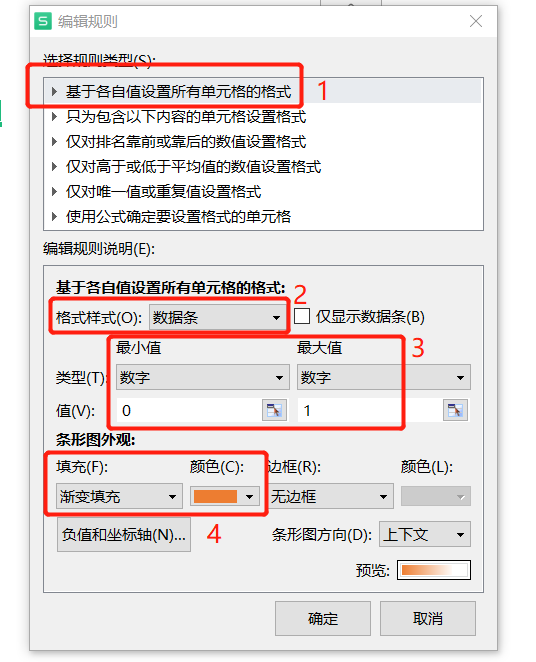## 8.图标指示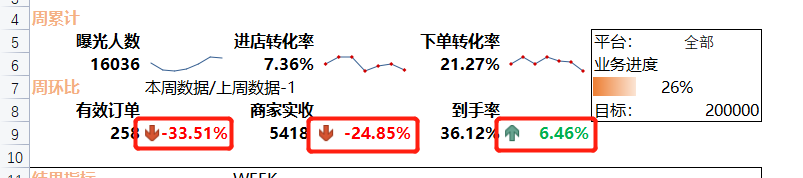为小看板中的周环比添加图标指示。正数显示为绿色，同时绿色箭头向下；负数或零时显示为红色，同时红色箭头向下。设置条件格式如下：
菜单栏【开始】→功能区【条件格式】→【新建规则】→选择规则类型【只包含一下内容的单元格设置格式】→【单元格值】【大于】【=0】→【格式】→【字体】→【颜色】

• 分别设置字体当数值大于0绿色、小于等于0黄色：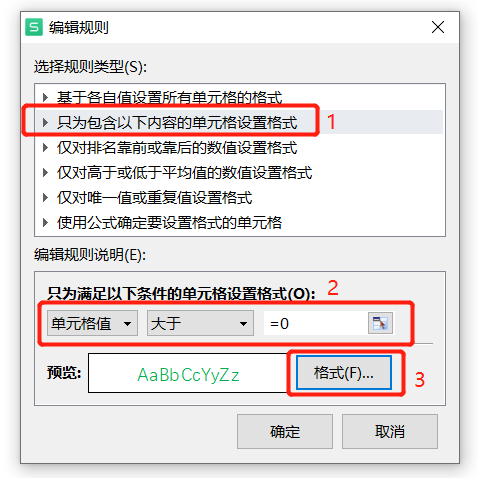• 设置图标
菜单栏【开始】→功能区【条件格式】→【新建规则】→格式样式【图标集】→选择对应图标→值【>=】【0】→类型【数字】→【确定】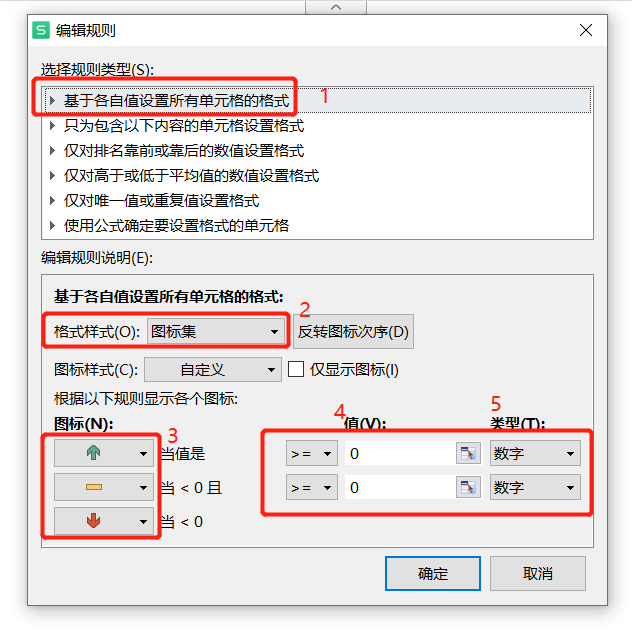所有条件设置如下，利用格式刷使该条件格式应用在其他环比数据上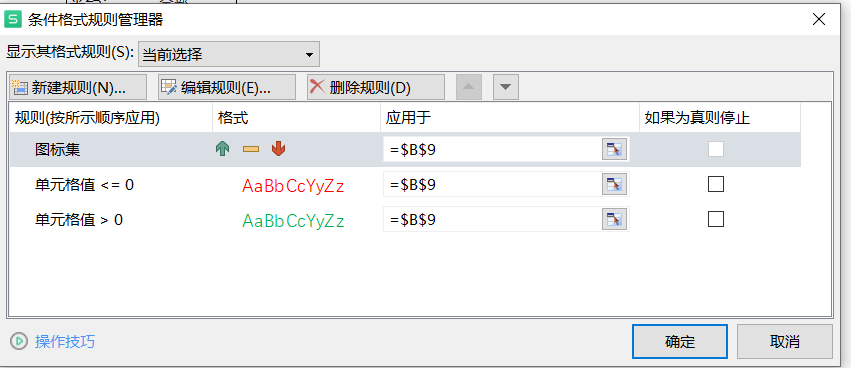## 9.突出显示

• 标记结果指标中GMV低于平均值的数据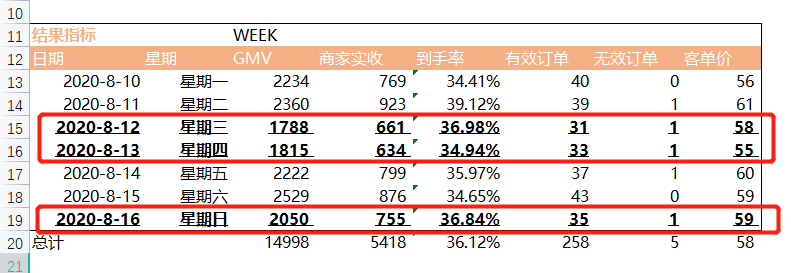选中条件判断区域→菜单栏【开始】→功能区【条件格式】→【新建规则】→选择规则类型【使用公式确定要设置的单元格】→设置公式，判断C13-C19整列数据→【格式】→【字体】→下划线【单下划线】→字形【粗体】→【确定】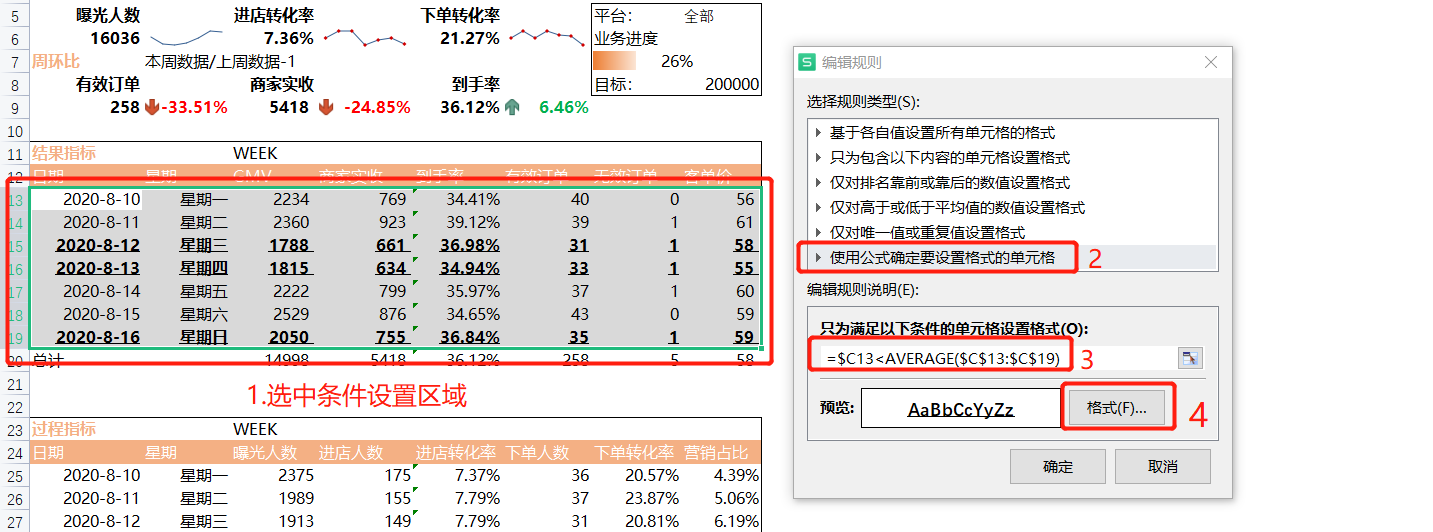## 10.细节美化

1)标题居中、字体加粗放大
2)关键字、关键数据加粗
3)表头添加背景颜色、修改字体颜色
4)添加外框线

展开全文经验分享
• 众所周知，中国式报表的特点就是格式复杂，信息量大。中国式报表的复杂大多都是表头比较复杂，三五层的大格嵌小格、带斜线的表头都很常见，所以中国式报表很少有不带格线的，如果没有格线的话很容易看走眼。中国式...

众所周知，中国式报表的特点就是格式复杂，信息量大。中国式报表的复杂大多都是表头比较复杂，三五层的大格嵌小格、带斜线的表头都很常见，所以中国式报表很少有不带格线的，如果没有格线的话很容易看走眼。中国式报表的信息量大，通常一张报表都会包含各种各样的数据，例如明细数据、汇总数据等，Smartbi提供了电子表格设计器工具，可以满足中国式报表的各种需求。

那电子表格是什么呢？

电子表格是企业报表平台的解决方案专家，它基于Excel创新地实现报表设计，可以满足各种格式的行业监管报表、内部管理报表的需求。由于本身Excel大家都非常熟悉，不像其他报表工具需要有专业的使用背景，或者专业的工程师提供支持，所以电子表格的学习成本是比较低的，调整报表样式也会比较简单方便。

二、安装插件

在使用电子表格设计器做报表前，需要先安装一个Excel插件，安装Excel插件可以将Smartbi的报表资源添加到Excel/WPS表格中，进而可以在Excel/WPS表格中引用Smartbi中的资源，生成电子表格报表。

Excel插件安装简单，与Excel、WPS表格兼容良好，使用方便，Excel插件

那么我们在安装完Excel插件后，可以看到在Excel工具栏会多出来一个Smartbi的页签，此时我们可以点击工具栏上的 设置 按钮，在“服务器设置”界面填入Smartbi服务器URL、登录用户名和密码，然后点击 确定 按钮即可登录。电子表格

三、界面介绍

电子表格的界面主要分为三部分：工具栏、报表设计区、数据集面板。

工具栏：分类存放电子表格特殊功能快捷键，如报表操作、参数设置等。

报表设计区：用于对电子表格进行设计，包括表头设计、表格设计、控件设计等。

数据集面板：用于展示电子表格可用的资源，包含关系数据源、数据集、透视分析。电子表格

四、报表制作

那么接下来院长就带着大家一起基于电子表格来制作一张交叉报表，制作流程如下：第一步：创建关系数据源

创建与业务数据库的连接，获取业务数据库相关资源。

入口：在系统主界面点击数据连接，然后在数据库选择界面切换到关系数据库，点击对应的数据库类型弹出数据源创建界面。第二步：创建数据集

基于创建的关系数据源创建数据集，获取报表设计需要的字段，形成数据的集合。

入口：在系统主界面点击数据准备，选择数据集，在对应的目录节点上右键新建数据集，选择原生SQL数据集，数据来源选择上述数据源，写入SQL语句。第三步：表样设计

1. 在报表设计区设计好静态表样，包含合并单元格、边框设置和颜色填充等；

2. 设置斜线表头，点击对应的合并单元格，将工具栏上的格式更改为斜线，填写相应的显示内容，分区内容用“|”隔开，报表发布后会自动转换。第四步：拖拽字段

将对应数据集的字段拖拽到设计好的表样中，并且修改字段相应的单元格属性。

设置区域和城市字段为从上到下扩展分组显示，产品大类名称和产品名称从左到右扩展分组显示，销售量为不扩展汇总求和，并且设置数据为空时显示为0。第五步：发布预览

最后，将设计的好报表发布到服务器，我们可以在设计器中直接预览。展开全文•java poi
• 本程序是java程序 直接将DoExcelHelper.java放入到项目中修改下package的名就行了。 怎么样使用DoExcelHelper这个类： 具体的请看DoExcelHelper.java...setTitle(String)方法是添加报表最顶部的标题的。 setF_name(Strijava 合并
• 如何使用第三方免费Excel插件来制作Excel报表控件 数据
• 今天就带大家来实战一波，使用Python自动化生成数据报表！从一条条的数据中，创建出一张数据报表，得出你想要的东西，提高效率。主要使用到pandas、xlwings以及matplotlib...
• 工程目录结构以及所需的jar包如下：工具：poi-2.0-RC2-20040102.jar(注意：这里并没有使用本机自带的Excel2007制作模板)现在很多工具都要版本对应才能使用,eg:在使用poi-2.0-RC2时 必须使用老版本的Excel新建xls文件...
• 利用excel中的数据源制作数据地图的方法以不新奇，可以直接利用excel制作，也可以利用插件和其他软件来实现。本文就从这三方面来谈谈如何利用制作数据源地图。数据
• 我是小z今天给大家分享一篇俊红新书《对比Excel，轻松学习Python报表自动化》中关于报表自动化实战的内容，文末也会免费赠送几本新书。这本书是俊红新作，强烈安利！这篇文章将带你了解报表自动化的流程，并教你用...python 自动化办公
• 在老师的日常工作中对学生的成绩进行统计分析管理是一项非常重要也是十分...报表制作各科统计分析图表等如果能够利用EXCEL强大的数据处理功能就可以让各位老师迅速完成对学生的成绩的各项分析统计工作下面就向各位老师
• Smartbi Insight以中国式报表、多维度分析、可视化仪表盘为核心，满足报表需求的功能，以“真Excel”为特色，将Office Excel和WPS表格变为企业级WEB报表设计器，表格样式与计算逻辑的灵活性不言而喻。大数据
• 下图是Excel的中各个部分的组成关系，我们工作中每天会处理很多Excel文件，一个Excel文件其实就是一个工作簿。你在每次新建一个Excel文件时，文件名都会默认是工作簿x，其中x就是你新建的文件个数。而一个工作簿里面...算法 人工智能 python
• 企业报表是否能反映真实情况？财务总监是否能随时监控企业报表？IT与业务人员的财务配合模式，是否应该改变？ 目前财务分析，大部分的使用工具是ExcelExcel作为大部分公司财务分析人员最经常用到的软件，确实有着...财务分析 大数据
• 如何利用勤哲Excel服务器制作库存管理系统一、系统框架：整个系统分为四部分：基础数据，进货管理，出货管理，统计报表。其中商品的进出库采用先进先出的方法，出库价及成本价采用移动加权平均算法。二、各模块功能...
• Excel在工业报表制作中的应用 工业控制中接触到大量的专用工业软件,在生成报表时因为使用者对各类软件习惯不同,在应用时 造成诸多不便。同时各种专用软件报表格式相对固定,不能完全适合各类企业对生产报表的要求。针...
• 我们小组上个季度接手了一个项目其中需要实现创建excel文档的功能，寻找实现这个功能的控件的任务分配给了我，通过百度搜索我找到了一个免费的控件，它是由E-iceblue公司推出的spire.xls控件，接下来的一段时间我...
• 面对“报表”，我们“跑不掉”～本文通过使用Excel+Python，梳理制作一份基础的日报流程：1、制作报表前“思考人生“2、取数，制作原始数据表3、调用，制作中间数据表4、自动化生成Excel日报5、...
• 制作报表画面>5.1 控件添加5.2 脚本6 <运行结果> 1 <概述> 在工业生产中报表一直占有非常重要的部分，它一般用来记录现场的工艺参数、能耗信息和统计信息，作为分析企业运营情况的依据。 本文以Wincc...
• 日常工作中，经常需要制作excel报表，并通过邮件发送出去。 【设计思路】 1、利用Python生成excel及发送邮件； 2、建立一张邮件发送配置表，用来配置所有需要发送的邮件基本信息；再建立一张邮件发送内容表，通过...python 发送邮件
• Access利用excel制作复杂报表的方法 在读这篇文章以前首先要提醒大家，Access 本身的报表也具有很强的实用性和强大的功能，只有当你发掘了其本身全部的功能却仍不能满足你对报表的特殊要求时才请使用 Excel 输出...
• 利用模板语法快速生成 Excel 报表》的技术分享。 本期课程，我们将借助服务端表格组件GcExcel 的模板语法来完成Excel报表的生成，通过使用简单的语法标记，可以在不借助任何第三方组件的情况下，结合数据源，自动化...
• 1. 单击“添加引用”对话框的...注意：Microsoft Excel 11 Object Library中Excel 11 的版本与安装的OFFICE版本有关。 在编程之前还需要引入命名空间using Microsoft.Office.Interop.Excel; 注意：有的机器上需要用...